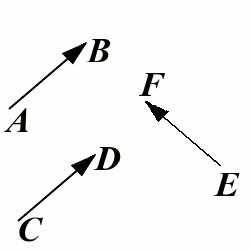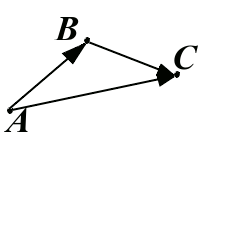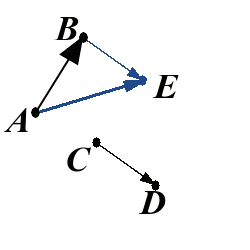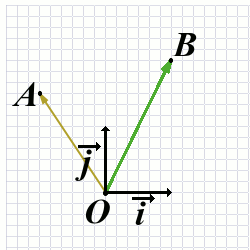# Vectors. Equations of Lines and Planes

## Introduction

Vectors are main objects of study in multivariable calculus. They have the same role that numbers have in single-variable calculus. It is very important to gain a solid understanding of vectors before proceeding to multivariable calculus. Please read this section carefully, and make sure you are able to solve most of the exercises in quizzes in the bottom of this page.

## Vectors: Geometric point of view

Before we can see why vectors may be useful in calculus, let us review our former knowledge about main properties of vectors.

Vector: A wrong definition Vector is a line segment with an arrow on one of its ends.

If you don’t like the previous definition, you are right, and you should be proud. But we won’t provide a better one in this page. This website does have (or will have) a precise definition of the vector in the appropriate section. Here we will just review how vectors work.

### Equal vectors

Two vectors are equal if they lie on parallel lines, have the same direction, and have the same magnitude.In the picture above, we have $$\overrightarrow{AB}=\overrightarrow{CD}$$, while $$\overrightarrow{AB}\neq \overrightarrow{EF}$$.

You may have noticed that the vectors $$\overrightarrow{AB}$$ and $$\overrightarrow{EF}$$ have the same length. That’s right.

We write $$|\overrightarrow{AB}|=|\overrightarrow{EF}|$$ to say that the vectors $$\overrightarrow{AB}$$ and $$\overrightarrow{EF}$$ have the same length.

Adding two vectors is a geometric operation that produces a vector.If one vector starts exactly where the other ends, then the addition is easy. This case is illustrated by the picture above. We have $$\overrightarrow{AB}+\overrightarrow{BC}=\overrightarrow{AC}$$.In the example from the picture above, we want to find $$\overrightarrow{AB}+\overrightarrow{CD}$$. These two vectors are drawn in black. We will reduce this case to the previous one in which one vector starts where the other ends. We first construct a point $$E$$ such that $$\overrightarrow{CD}=\overrightarrow{BE}$$. Then we have

$\overrightarrow{AB}+\overrightarrow{CD}=\overrightarrow{AB}+\overrightarrow{BE}=\overrightarrow{AE}.$

The point $$E$$ is blue in the picture, as well as the vector $$\overrightarrow{BE}$$. The resulting vector $$\overrightarrow{AE}$$ is also blue.

The opposite vector to the vector $$\overrightarrow{AB}$$ is the vector $$\overrightarrow{BA}$$. We also write

$-\overrightarrow{AB}=\overrightarrow{BA}.$

Now we can define subtraction of vectors: $$\overrightarrow{AB}-\overrightarrow{CD}=\overrightarrow{AB}+(-\overrightarrow{CD})=\overrightarrow{AB}+\overrightarrow{DC}$$.

### Multiplying vector by a scalar

The vector $$2\overrightarrow{AB}$$ is the vector that is parallel to $$\overrightarrow{AB}$$, has the same direction, but is twice the length of $$\overrightarrow{AB}$$. Similarly, we define $$3\overrightarrow{AB}$$, and $$\pi\overrightarrow{AB}$$.

## Vectors: Algebraic point of view

This is the beginning of the multivariable calculus. We will start by encoding vectors in a plane using two variables.

### Two dimensions

When we say two dimensions, we mean that we are dealing with a plane. Let us choose one point in the plane and denote it by O. This point will be called the origin. Let us place two vectors of length 1 that start at O and are perpendicular to each other. Let us call them $$\overrightarrow i$$ and $$\overrightarrow j$$. Then for each point $$A$$ in the plane we can express $$\overrightarrow{OA}$$ using only vectors $$i$$ and $$j$$.In the previous picture we can represent $$\overrightarrow{OB}=\overrightarrow{i}+2\overrightarrow{j}$$, and $$\overrightarrow{OA}=-\overrightarrow{i}+1.5\overrightarrow{j}$$. We also say that each vector is a linear combination of $$\overrightarrow i$$ and $$\overrightarrow j$$.

Each vector in the plane can be written in terms of $$\overrightarrow{i}$$ and $$\overrightarrow{j}$$. We can write

$\overrightarrow{AB}=\overrightarrow{AO}+\overrightarrow{OB}=-\overrightarrow{OA}+\overrightarrow{OB}=\overrightarrow{i}-1.5\overrightarrow{j}+\overrightarrow{i}+2\overrightarrow{j}=2\overrightarrow i+0.5\overrightarrow j.$

We say that 2 and 0.5 are coordinates of the vector $$\overrightarrow{AB}$$ and instead of $$\overrightarrow{AB}=2\overrightarrow i+0.5\overrightarrow j$$ we will often write $$\overrightarrow{AB}=\langle 2,0.5\rangle$$. Similarly, $$\overrightarrow{OA}=\langle -1,1.5\rangle$$ and $$\overrightarrow{OB}=\langle 1,2\rangle$$.

## Vectors in three dimensions

The story is exactly the same. We fix one point, O and three unit vectors $$\overrightarrow i$$, $$\overrightarrow j$$, and $$\overrightarrow k$$. We can express each vectors as a linear combination of $$\overrightarrow i$$, $$\overrightarrow j$$, and $$\overrightarrow k$$. We also write vectors in coordinate notation, so that $$\langle 3, 5, -2\rangle$$ represents the same vector as $$3\overrightarrow i+5\overrightarrow j-2\overrightarrow k$$.

## Dot product of vectors

If two vectors are written in their coordinate notation, $$\overrightarrow u=\langle u_x,u_y,u_z\rangle$$ and $$\overrightarrow v=\langle v_x, v_y,v_z\rangle$$ we define the scalar product (often called the dot product) of vectors $$\overrightarrow u$$ and $$\overrightarrow v$$ in the following way:

$\overrightarrow u\cdot\overrightarrow v=u_xv_x+u_yv_y+u_zv_z.$

The dot product of two vectors is always a scalar. For example, $$\langle 3,2,-1\rangle\cdot \langle -4,5,2\rangle=3\cdot (-4)+2\cdot 5+(-1)\cdot 2=-4$$.

The following properties of the dot product are very easy to verify. However, they will have powerful consequences:

Theorem (Properties of dot product) $\overrightarrow u\cdot\overrightarrow v=\overrightarrow v\cdot\overrightarrow u;$ $\overrightarrow u\cdot \overrightarrow u=|\overrightarrow u|\geq 0;$ $\overrightarrow u\cdot (\alpha \overrightarrow v)=(\alpha\overrightarrow u)\cdot \overrightarrow v=\alpha (\overrightarrow u\cdot\overrightarrow v);$ $\overrightarrow u\cdot(\overrightarrow v+\overrightarrow w)=\overrightarrow u\cdot\overrightarrow v+\overrightarrow u\cdot\overrightarrow w.$

Theorem (Cosine theorem) Denote by $$\theta$$ the angle between vector $$\overrightarrow u$$ and $$\overrightarrow v$$ (measured once the vectors are placed to have the same starting point). Then we have

$\overrightarrow u\cdot \overrightarrow v=|\overrightarrow u|\cdot|\overrightarrow v|\cos\theta.$

## Cross product

We will start by formal definition of the cross product.

Definition (Cross product) If $$\overrightarrow u=\langle x_u,y_u,v_u\rangle$$ and $$\overrightarrow v=\langle x_v,y_v,z_v\rangle$$ are two vectors, then their cross product $$\overrightarrow u\times \overrightarrow v$$ is defined as the following vector: $\overrightarrow u\times\overrightarrow v=\langle y_uz_v-y_vz_u,-x_uz_v+x_vz_u,x_uy_v-x_vy_u\rangle.$

It is easier to remember the cross product if it is written in the form of a determinant: $\overrightarrow u\times\overrightarrow v=\mbox{det }\left|\begin{array}{ccc} \overrightarrow i&\overrightarrow j&\overrightarrow k\newline x_u&y_u&z_u\newline x_v&y_v&z_v\end{array}\right|.$

The following theorem lists the properties of the cross product. It is very easy to prove (using just the definition of the cross product, and following the order in which the statements are listed).

Theorem (Properties of cross product) Assume that $$\overrightarrow u$$, $$\overrightarrow v$$, and $$\overrightarrow w$$ are three vectors, and $$\alpha$$, $$\beta$$, and $$\gamma$$ are three scalars. Then:

• $$\overrightarrow u\times (\beta\overrightarrow v+\gamma\overrightarrow w)=\beta\overrightarrow u\times\overrightarrow v+\gamma\overrightarrow u\times\overrightarrow w$$.

• $$\overrightarrow u\times\overrightarrow v=-\overrightarrow v\times\overrightarrow u$$

• $$\left|\overrightarrow u\times\overrightarrow v\right|^2+\left(\overrightarrow u\cdot\overrightarrow v\right)^2=\left|\overrightarrow u\right|^2\cdot\left|\overrightarrow v\right|^2$$

• $$\left|\overrightarrow u\times\overrightarrow v\right|=\left|\overrightarrow u\right|\cdot \left|\overrightarrow v\right|\cdot \left|\sin \theta\right|$$, where $$\theta$$ is the angle between vectors $$\overrightarrow u$$ and $$\overrightarrow v$$. This implies that the length of the cross product is equal to the area of the parallelogram determined by $$\overrightarrow u$$ and $$\overrightarrow v$$.

• The vector $$\overrightarrow u\times \overrightarrow v$$ is perpendicular to both $$\overrightarrow u$$ and $$\overrightarrow v$$.

## Triple product

Definition (Triple product) If $$\overrightarrow u$$, $$\overrightarrow v$$, and $$\overrightarrow w$$ are three vectors, then we define their triple product as $\left[\overrightarrow u, \overrightarrow v, \overrightarrow w\right]=\overrightarrow u\cdot \left(\overrightarrow v\times\overrightarrow w\right).$

Notice that the result of a triple product is a scalar, and the absolute value of that scalar is equal to the volume of the parallelepiped spanned by the vectors $$\overrightarrow u$$, $$\overrightarrow v$$, and $$\overrightarrow w$$.

## Equations of lines

You are probably familiar with the equations of the form $$y=3x+5$$. There are many ordered pairs $$(x,y)$$ of real numbers. Some of them satisfy the previous equation, others don’t. Those that do satisfy the equation determine the points in the plane - and if you paint those points in green - you get a green line.

This is the basic principle behind equations of geometric objects. A line in geometry is uniquely determined by a point and a vector. Let us consider a line $$\ell$$ that is determined by a point $$A(x_0,y_0,z_0)$$ and a vector $$v=\langle a,b,c\rangle$$. We want to describe all those points $$P(x,y,z)$$ that belong to $$l$$. Geometric description is easy:

The point $$P(x,y,z)$$ belongs to $$\ell$$ if and only if $$\overrightarrow {AP}$$ is parallel to $$\overrightarrow v$$.

The last sentence has alternative form:

The point $$P(x,y,z)$$ belongs to the line $$\ell$$ if and only if there exists a real number $$t$$ such that $$\overrightarrow {AP}=t\cdot \overrightarrow v$$.

Let us denote the origin by $$O$$. Notice that $\overrightarrow{AP}=\overrightarrow{AO}+\overrightarrow{OP}=\overrightarrow{OP}-\overrightarrow{OA}=\langle x,y,z\rangle-\langle x_0,y_0,z_0\rangle=\langle x-x_0,y-y_0,z-z_0\rangle.$ The last sentence can now be easily translated into algebraic form:

The point $$P(x,y,z)$$ belongs to the line $$\ell$$ if and only if there exists a real number $$t$$ such that $$\langle x-x_0,y-y_0,z-z_0\rangle=t\langle a,b,c\rangle$$.

The last form of the equation of the line is called the vector equation of the line $$\ell$$. Its equivalent version is used most often and is called the parametric equation of the line: $x=x_0+at$ $y=y_0+bt$ $z=z_0+bt$ $t\in\mathbb R.$

## Equations of planes

A plane in three dimensional space is uniquely determined by one of the following three combinations :

• (i) any three of its points
• (ii) a point and any two vectors
• (iii)a point and a normal vector

It turns out that in practice we use (ii) and (iii) to determine the equations of the plane. If we are given three points, we transform the problem from (i) to either (ii) or (iii) and try to deal with consequences.

### Case (ii): Parametric equations

Let us assume that we are given a point $$A$$ and two vectors $$\overrightarrow u=\langle a_u,b_u,c_u\rangle$$ and $$\overrightarrow v=\langle a_v,b_v,c_v\rangle$$. Let us consider the plane $$\alpha$$ that passes through $$A$$ and is parallel to both $$\overrightarrow u$$ and $$\overrightarrow v$$. A points $$P(x,y,z)$$ belongs to $$\alpha$$ if and only if there are two real numbers $$s$$ and $$t$$ such that $$\overrightarrow{AP}= s\overrightarrow u+t\overrightarrow v$$, i.e. $$\overrightarrow{OP}=\overrightarrow{OA}+s\overrightarrow u+t\overrightarrow v$$. This can be written as $x=x_0+sa_u+ta_v$ $y=y_0+sb_u+tb_v$ $z=z_0+sc_u+tc_v$ $s\in\mathbb R, \;\;\; t\in\mathbb R.$ The above equations are called parametric equations of the plane $$\alpha$$.

Notice that the parametric equations of the line have one parameter, while the parametric equations of the plane have two parameters ($$s$$ and $$t$$). That is why people say that line has dimension 1, while plane has dimension 2.

### Case (iii): Symmetric equations

Assume we are given a point $$A(x_0,y_0,z_0)$$ and a vector $$\overrightarrow n=\langle a,b,c\rangle$$. Let us find the equation of the plane $$\alpha$$ that passes through $$A$$ and is orthogonal to $$\overrightarrow n$$. A point $$P(x,y,z)$$ belongs to $$\alpha$$ if and only if the vector $$\overrightarrow{AP}$$ is orthogonal to $$\overrightarrow n$$, i.e. if and only if $$\overrightarrow{AP}\cdot \overrightarrow n=0$$. Since $$\overrightarrow{AP}=\langle x-x_0, y-y_0, z-z_0\rangle$$, the last statment can be written as $(x,y,z)\in\alpha\;\;\;\Leftrightarrow \;\;\; \langle x-x_0,y-y_0,z-z_0\rangle \cdot \langle a,b,c\rangle=0,\;\;\;\mbox{ or, equivalently}$ $a(x-x_0)+b(y-y_0)+c(z-z_0)=0.$ Sometimes the last equation is written as $$ax+by+cz=d$$, where $$d=ax_0+by_0+cz_0$$ is a constant.

Theorem (Distance between a point and a plane) Assume that $$A(x_1,y_1,z_1)$$ is a point, and $$\alpha$$ is a plane given by $a(x-x_0)+b(y-y_0)+c(z-z_0)=0.$ Then the distance between the point $$A$$ and the plane $$\alpha$$ is given by $\mbox{dist }(A,\alpha)=\frac{\left|a(x_1-x_0)+b(y_1-y_0)+c(z_1-z_0)\right|}{\sqrt{a^2+b^2+c^2}}.$

Example A plane is given by its symmetric equation $$8x-7y+5z=21$$. Find the point on the plane that is closest to the point $$P$$ with coordinates $$(3,1,-2)$$.

## Practice problems

Problem 1. If $$A=(0,3,1)$$, $$B=(2,1,1)$$, and $$C=(1,4,-1)$$ find the dot product $$\overrightarrow{AB}\cdot\overrightarrow{CB}$$.

Problem 2. Find the distance between the point $$A(3,1,0)$$ and the plane determined by the points: $$B(0,1,2)$$, $$C(-1,3,1)$$, and $$D(3,-2,-1)$$.

Problem 3. Assume that $$A(1,5,2)$$, $$B(-3,4,-1)$$, and $$C(0,-2,1)$$ are vertices of the triangle. Let $$M$$ be the midpoint of the segment $$AB$$. Determine $$\overrightarrow{AB}\cdot \overrightarrow{CM}$$.

Problem 4. Consider the following six points: $A(3,1,-2),\;\;\; B(-2,1,-5),\;\;\; C(3,0,4),$ $D(1,0,-1),\;\;\; E(-4,0,1),\;\;\; F(6,0,7).$ Determine which of the following statements is true.
• (A) All six points belong to a plane.
• (B) None four of the given points belong to a plane.
• (C) The points $$A$$, $$B$$, $$E$$, and $$F$$ belong to a plane.
• (D) The points $$A$$, $$B$$, $$D$$, and $$E$$ belong to a plane.
• (E) The points $$A$$, $$B$$, $$C$$, and $$F$$ belong to a plane.

Problem 5. Consider the points $$O(0,0,0)$$, $$A(3,-1,0)$$, $$B(-1,2,-3)$$, and $$C(-2,1,2)$$. Find the shortest distance between the lines $$OA$$ and $$BC$$.